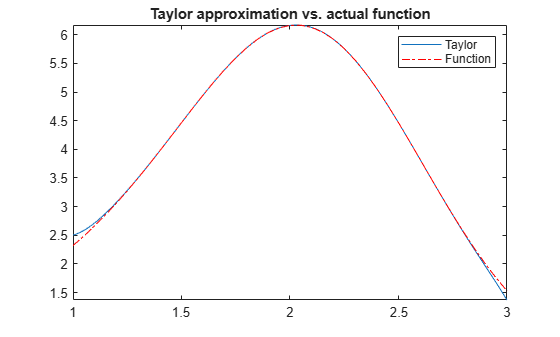Taylor Series

The statements

syms x
f = 1/(5 + 4*cos(x));
T = taylor(f, 'Order', 8)

return

T =
(49*x^6)/131220 + (5*x^4)/1458 + (2*x^2)/81 + 1/9

which is all the terms up to, but not including, order eight in the Taylor series for f(x):

$\sum _{n=0}^{\infty }{\left(x-a\right)}^{n}\frac{{f}^{\left(n\right)}\left(a\right)}{n!}.$

Technically, T is a Maclaurin series, since its expansion point is a = 0.

These commands

syms x
g = exp(x*sin(x));
t = taylor(g, 'ExpansionPoint', 2, 'Order', 12);

generate the first 12 nonzero terms of the Taylor series for g about x = 2.

t is a large expression; enter

size(char(t))
ans =
1       99791

to find that t has about 100,000 characters in its printed form. In order to proceed with using t, first simplify its presentation:

t = simplify(t);
size(char(t))
ans =
1        6988

Next, plot these functions together to see how well this Taylor approximation compares to the actual function g:

xd = 1:0.05:3;
yd = subs(g,x,xd);
fplot(t, [1, 3])
hold on
plot(xd, yd, 'r-.')
title('Taylor approximation vs. actual function')
legend('Taylor','Function')Special thanks is given to Professor Gunnar Bäckstrøm of UMEA in Sweden for this example.

Symbolic Math Toolbox Documentation

Mathematical Modeling with Symbolic Math Toolbox

Get examples and videos